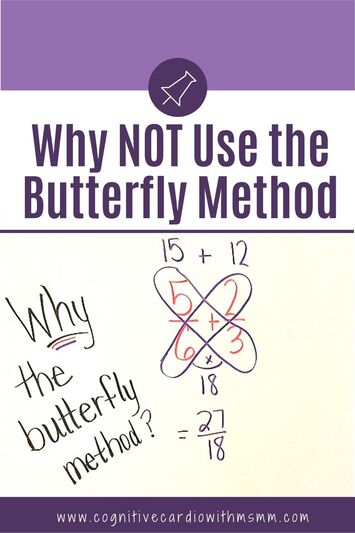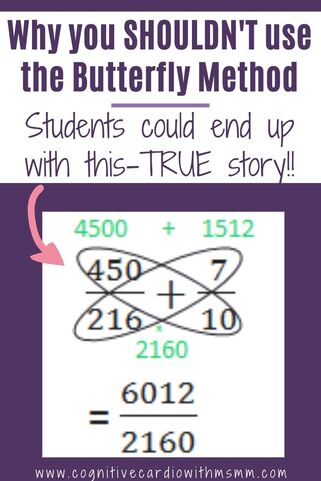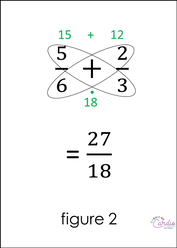# WHY NOT the Butterfly Method When Adding and Subtracting Fractions?

## Why You Shouldn’t Use the Butterfly Method​The “butterfly method” for comparing, adding, and subtracting fractions – WHY teach this? This is a serious question….why is this being taught?

​I don’t understand why the “butterfly method” is being used….

I understand that it works and that it can make finding an answer easier for students (for simple fractions). However, I’ve found definite drawbacks to this method, so I don’t like to see students adding and subtracting fractions this way!

Let me explain what I’ve seen in 6th grade math:

## Butterfly Method for Comparing Fractions

In past years, students would come to 6th grade, having learned the butterfly method for comparing fractions…..

• they often had no idea why it worked
• they knew the trick better than they knew how to find a common denominator
• they didn’t seem to understand that the products from the cross-multiplying were the actual numerators they would get if they made certain equivalent fractions (shown in figure 1 below)

They just knew it worked.

This bothered me, because I believe they should understand why things work. So I always made sure to explain why the method worked.

## Butterfly Method for Adding and Subtracting FractionsBut this year, I had 6th grade math students tell me they were taught to use the butterfly method to add and subtract fractions (cross multiply and add those products, then multiply the denominators together – shown in figure 2).

But again, they didn’t really have a conceptual
understanding of WHY it works.

It seems that many students are being taught “tricks” like this, to make learning fraction operations “easier and fun.”

In reality, students aren’t learning what it means to add or subtract fractions. (And, really, why wouldn’t we want them to see that 6 is the LCD in the problem in figure 2? Why would we want to them to use a larger denominator and then have to do more simplifying to lowest terms??)

## Using the Butterfly Method on Larger Fractions:I recently gave students problem solving that required them to use all fraction operations. Since adding and subtracting is in the 5th grade math curriculum (and I teach 6th), I did just a brief review of adding and subtracting fractions before students worked on these problems – to see what they remembered.
​This is when I found out that many of them had been taught the butterfly method, among others.

In the problem solving, students had to add 5/6, 2/3, 7/12, and 7/10.
And here’s where the butterfly method totally fails the students who have learned to rely on it, not only because they don’t understand why it works, but also because it becomes so cumbersome!

They couldn’t use the butterfly method to add 3 or 4 fractions at a time, so they added two fractions at a time. Instead of finding a common denominator for all 4 fractions, they found a new common denominator each time they added on the next fraction (and those denominators sure weren’t the LCDs!).

• For example, they added 5/6 and 2/3, getting a denominator of 18, as in figure 2.
• Then they added 27/18 and 7/12, as in figure 3,  getting a denominator of 216.
• From there, they added 450/216 and 7/10, shown in figure 4. They ended up with a HUGE denominator that they then had to work really hard to simplify!

It might seem surprising that they continued this process to get such huge numbers, but some of them did, because this was the method they learned and depended on. I was so shocked to see this….and this is the first year I saw this method used in this way.

## Do Math “Tricks” Really Help?

When we as teachers (or parents) find certain tricks that work for simple math problems, we need to look ahead to what our students will experience in future years.

We need to try these methods with more complicated problems, to see if they will still be effective.

We need to think about whether the “tricks” teach them math concepts, or number sense, or number connections….or do they just teach short-cuts?

I have no problem with teaching short-cuts once the conceptual understanding is there. But short-cuts before understanding is detrimental to our students. They are capable of understanding the concepts and we need to have faith that they can “get it” without the tricks.

Thanks for reading! What are your thoughts or experiences with the “butterfly method”?

​Check out the Fractions: From Foundations to Operations program.
 Need some materials to supplement your fraction instruction? Grab this free fraction toolkit when you join my email community!

## EllieWelcome to Cognitive Cardio Math! I’m Ellie, a wife, mom, grandma, and dog ‘mom,’ and I’ve spent just about my whole life in school! With nearly 30 years in education, I’ve taught:

• All subject areas in 4th and 5th grades
• Math, ELA, and science in 6th grade (middle school)

I’ve been creating resources for teachers since 2012 and have worked in the elearning industry for about five years as well!